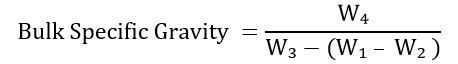## What is Specific Gravity Test of Coarse Aggregate?

The test specific gravity of coarse aggregate is used to calculate the specific gravity of a coarse aggregate sample. Specific gravity of coarse aggregate is determined by the ratio of weight of a given volume of aggregate sample to the weight of equal volume of water. This test is similar in nature to the specific gravity of fine aggregate test.

What is Specific Gravity of Aggregates?
The specific gravity of an aggregate is considered as the measure of strength or quality of the material. Specific gravity is defined as the ratio of weight of a given volume of aggregate to the weight of equal volume of water. Aggregates having low specific gravity are generally weaker than those with aggregates having high specific gravity. This property helps in a general identification of aggregates.

What is the Specification of Absorption of Aggregates?

Water absorption gives an idea on the internal structure of aggregate. Aggregates having more absorption are more porous in nature. These aggregates are generally considered unsuitable for construction, unless found to be acceptable based on strength, impact and hardness tests.Apparatus for specific gravity test of aggregate

# Specific Gravity and Water Absorption of Coarse Aggregate Lab Report

Hi friends, you are welcomed in the world of Civil Allied Gyan. Read here definition, apparatus, IS code, test procedure, formula, result and lab report of specific gravity and water absorption of coarse aggregate.

### Apparatus Required for Specific Gravity Test of Coarse Aggregate:-

• Wire Mesh Bucket
• Container for filling water and suspending the wire mesh basket in the water
• Airtight container of capacity similar to wire basket
• A shallow tray
• Two dry absorbent clothes
• Weighing Balance
• OvenApparatus for specific gravity test of aggregate

### IS Code of Specific Gravity Test of Coarse Aggregate:-

• IS: 2386 (Part 3): 1963, Methods of test for aggregates for concrete (Determination of Specific Gravity of aggregates)

### Procedure for Specific Gravity Test of Coarse Aggregate:-

1. Take clean sample of coarse aggregate (about 2 kg). The sample should be washed to remove fine aggregates. The aggregates coarser than 6.3 mm are taken.
2. Then place the aggregate sample in the wire basket.
3. Immerse the wire basket in distilled water, which is at a temperature of 22 ºC to 32 ºC.
4. Immediately after immersion, remove the entrapped air from the sample by lifting the basket 25 mm above the base of the tank and allow it to drop, 25 times at a rate of about one drop per second.
5. Keep the wire basket with aggregates sample completely immersed in water for a period of 24 ± 0.5 hours.
6. Weigh the wire basket and aggregates at the time they suspended in water, at a temperature of 22 ºC to 32 ºC (W g).
7. Weigh the wire basket in water (W g).
8. Remove the basket and aggregates from water and dry the aggregates with dry absorbent cloth.
9. Weigh the surface dried aggregates sample (W g).
10. Place the aggregates sample in a shallow tray and heat to about 110 ºC in the oven for 24 hours.
11. Later, cool the oven dried aggregates sample in an airtight container and weigh it (W g).

Observations:-
Table: Observation table for specific gravity and water absorption of coarse aggregate
 Description Observed values Weight of saturated aggregate and basket in water (W₁ g) Weight of basket in water (W₂ g) Weight of saturated aggregates in air (W₃ g) Weight of oven dry aggregates in air (W₄ g) Apparent Specific Gravity: W₄ / [W₄ – (W₁ - W₂ )] Bulk Specific Gravity:  W₄ / [W₃– (W₁ - W₂ )]

Calculations:-
Formula for specific gravity and water absorption of coarse aggregate:-Result:-
The specific gravity of coarse aggregate is =……………
The water absorption of coarse aggregate is =……………

General Remarks:-
1. The specific gravity of aggregates normally used in construction ranges from around 2.5 to 3.0 with an average value of about 2.68.
2. Specific gravity of aggregates is considered as a measurement of strength. Aggregates having higher specific gravity are generally considered as having higher strength and aggregates having low specific gravity are generally considered as having weaker strength.